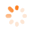# Using AggregateExpression and GridViewSummaryItem

GridViewTop achievementsRank 1
Brennan asked on 25 Nov 2011, 12:53 AM
Hey all,

I have run into an issue using AggregateExpressions with GridViewSummaryItems.

If I use the following method and Sum(Foo2) is 0, I will obviously get a Divide by Zero exception.  Is there anyway handle this when using AggregateExpressions or will I have to use the CustomSummaryItem method as described here?

```Dim summaryItem As New GridViewSummaryItem()
summaryItem.Name = "Foo"
summaryItem.AggregateExpression = "(Sum(Foo) / Sum(Foo2)"```

Too clarify, I have already got the desired output (percentage of the sums of two other columns in my grid) using the CustomSummaryItem, but I would rather use AggregateExpressions if possible as it's cleaner and requires less code.

Thanks!

## 6 Answers, 1 is accepted

0Alexander
Telerik teamanswered on 28 Nov 2011, 10:34 AM
Hello Brennan,

You can use the Immediate IF function to check for zero values in your expression:
`summaryItem.AggregateExpression = ``"Sum(ID) / (IIf(Sum(ID2) > 0, Sum(ID2), 1))"`

Kind regards,
Alexander
the Telerik team

0Brennan
Top achievementsRank 1
answered on 10 Mar 2015, 08:52 PM
Hi there,

Here is what I'm trying:

`Dim` `real ``As` `GridViewSummaryItem = ``New` `GridViewSummaryItem(``"Real"``, ``"{0:#,##;(#,##);#}"``, ``"IIf(Sum(Tariff) > 0, ((Sum(Tariff) + Sum(Adj)) / Sum(Tariff)), 0) * 100"``)`

However, I am still getting a Divide By Zero exception.  I assume that this is because IIf() is evaluating both sides of the if statement regardless if Sum(Tariff) > 0 or not.  Is there any way around this?

Thanks,
0Brennan
Top achievementsRank 1
answered on 11 Mar 2015, 02:53 PM
Hi there,

Here is the work around I am using, which I am not proud of lol.

`Dim` `real ``As` `GridViewSummaryItem = ``New` `GridViewSummaryItem(``"Real"``, ``"{0:#,##;(#,##);#}"``, ``"IIf(Sum(Tariff) = 0, 0, ((Sum(Tariff) + Sum(Adj)) / (Sum(Tariff) + 0.000000001))) * 100"``)`

Please note I have also tried handling GroupSummaryEvaluate and testing for zero, but for this specific scenario, I need the exact formula I am using to be in a single GridViewSummaryItem as I call summaryItem.GetSummaryExpression() elsewhere in my code (I have implemented my own GridGroupContentCellElement to have aggregate values in the group rows) and need the full formula to be returned.
0Hristo
Telerik teamanswered on 13 Mar 2015, 02:41 PM
Hi Brennan,

Thank you for writing.

I tested your code and to me it appears that you add this really small number in the case where the sum of the Tariff column is greater than 0. ​Could you please try the following aggregate expression and let us know if it is ok for your project:
`Dim ``real1 ``As ``New ``GridViewSummaryItem(``"Real"``, ``"{0:#,##;(#,##);#}"``, ``"IIf(Sum(Tariff) = 0, 0, ((Sum(Tariff) + Sum(Adj)) / Sum(Tariff))) * 100"``)`

Regards,
Hristo Merdjanov
Telerik

Check out the Telerik Platform - the only platform that combines a rich set of UI tools with powerful cloud services to develop web, hybrid and native mobile apps.

0Brennan
Top achievementsRank 1
answered on 13 Mar 2015, 02:53 PM
Hi Hristo,

This is what I tried in my post, two posts up and it doesn't work.  It seems that IIf() calculates both branches of the IIf() statement regardless if tariff is zero or not, always leading to a divide by 0.  This is why I added the small number to tariff - to avoid the divide by zero exception when the IIf is calculating the logical branch that won't be used.
0Hristo
Telerik teamanswered on 18 Mar 2015, 08:04 AM
Hi Brennan,

Thank you for writing back.

After being read the aggregate expression is parsed. When there is an if statement, you would need to provide two values or expressions which will be considered for the different cases. If the if statement evaluates to true the first one will be passed otherwise the second. Please try the code snippet below:
`Public ``Class ``Form1`
`    ``Sub ``New``()`
`        ``InitializeComponent()`

`        ``Dim ``dataTable ``As ``New ``DataTable()`
`        ``dataTable.Columns.Add(``"Real"``, ``GetType``(``Integer``))`
`        ``dataTable.Columns.Add(``"Tariff"``, ``GetType``(``Integer``))`
`        ``dataTable.Columns.Add(``"Adj"``, ``GetType``(``Integer``))`

`        ``For ``i ``As ``Integer ``= 0 ``To ``9`
`            ``'dataTable.Rows.Add(i, i, i + 20)`
`            ``dataTable.Rows.Add(i, 0, i + 20)`
`        ``Next`

`        ``Me``.RadGridView1.DataSource = dataTable`
`        ``Me``.RadGridView1.AutoSizeColumnsMode = GridViewAutoSizeColumnsMode.Fill`

`        ``Dim ``real ``As ``New ``GridViewSummaryItem()`
`        ``real.Name = ``"Real"`
`        ``'real4.FormatString = "{0:#,##;(#,##);#}"`
`        ``Dim ``expr ``As ``String ``= ``"(IIf(Sum(Tariff) = 0, 0, ((Sum(Tariff) + Sum(Adj)) / Sum(Tariff)) * 100))"`
`        ``real.AggregateExpression = expr`
`        ``Dim ``summaryRowItem ``As ``New ``GridViewSummaryRowItem(``New ``GridViewSummaryItem() {real})`

`        ``Me``.RadGridView1.SummaryRowsTop.Add(summaryRowItem)`
`    ``End ``Sub`
`End ``Class`

Other examples and detailed information you can find here. I am also sending you screenshots of the results on my side for both cases.

I hope this helps. Should you have further questions please do not hesitate to write back.

Regards,
Hristo Merdjanov
Telerik

Check out the Telerik Platform - the only platform that combines a rich set of UI tools with powerful cloud services to develop web, hybrid and native mobile apps.

Tags
GridViewBrennan
Top achievementsRank 1Alexander
Telerik teamBrennan
Top achievementsRank 1Hristo
Telerik team